# Mirror And Water Images

## 20 Questions MCQ Test Science Olympiad Class 4 | Mirror And Water Images

Description
Attempt Mirror And Water Images | 20 questions in 40 minutes | Mock test for Class 4 preparation | Free important questions MCQ to study Science Olympiad Class 4 for Class 4 Exam | Download free PDF with solutions
QUESTION: 1

Solution:
QUESTION: 2

Solution:
QUESTION: 3

### In each of the following questions you are given a combination of letters and/or numbers followed by four alternatives (1), (2), (3) and (4). Choose the alternative which closely resembles the mirror image of the given combination.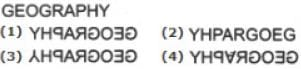Solution:
QUESTION: 4

In each of the following questions you are given a combination of letters and/or numbers followed by four alternatives (1), (2), (3) and (4). Choose the alternative which closely resembles the mirror image of the given combination.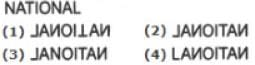Solution:
QUESTION: 5

In each of the following questions you are given a combination of letters and/or numbers followed by four alternatives (1), (2), (3) and (4). Choose the alternative which closely resembles the mirror image of the given combination.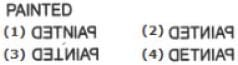Solution:
QUESTION: 6

Choose the correct mirror image of the given figure (X) from amongst the four alternatives.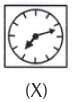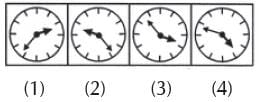Solution:
QUESTION: 7

Choose the correct mirror image of the given figure (X) from amongst the four alternatives.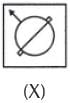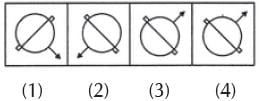Solution:
QUESTION: 8

Choose the correct mirror image of the given figure (X) from amongst the four alternatives.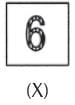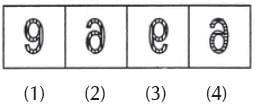Solution:
QUESTION: 9

Choose the correct mirror image of the given figure (X) from amongst the four alternatives.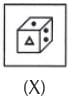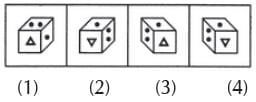Solution:
QUESTION: 10

Choose the correct mirror image of the given figure (X) from amongst the four alternatives.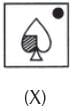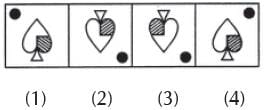Solution:
QUESTION: 11

Choose the correct mirror image of the given figure (X) from amongst the four alternatives.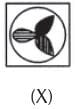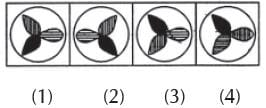Solution:
QUESTION: 12

Choose the correct mirror image of the given figure (X) from amongst the four alternatives.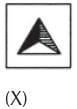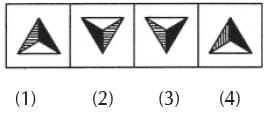Solution:
QUESTION: 13

Choose the correct water image of the given figure (X) from amongst the four alternatives.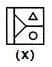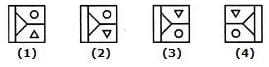Solution:
QUESTION: 14

Choose the correct water image of the given figure (X) from amongst the four alternatives.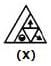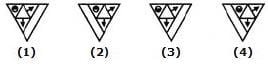Solution:
QUESTION: 15

Choose the correct water image of the given figure (X) from amongst the four alternatives.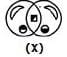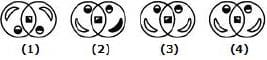Solution:
QUESTION: 16

Choose the correct water image of the given figure (X) from amongst the four alternatives.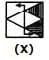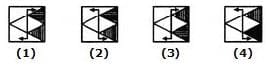Solution:
QUESTION: 17

Choose the correct water image of the given figure (X) from amongst the four alternatives.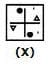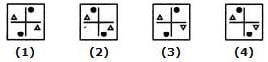Solution:
QUESTION: 18

Choose the correct mirror image of the given figure from amongst the four alternatives.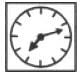Solution:
QUESTION: 19

Choose the alternative which closely resembles the mirror image of the given.
BRISK

Solution:
QUESTION: 20

Choose the alternative which closely resembles the mirror image of the given combination.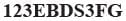Solution:Use Code STAYHOME200 and get INR 200 additional OFF Use Coupon Code